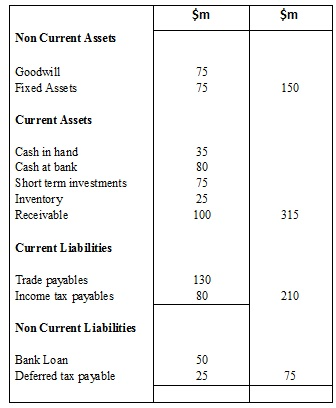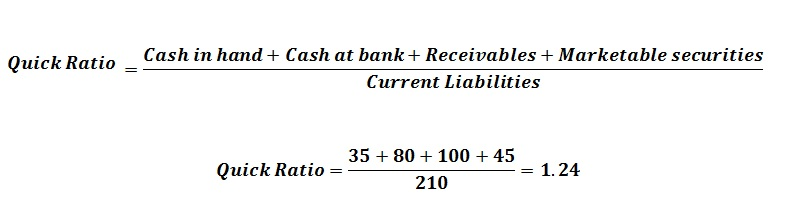# How to Calculate Acid Test Ratio

Acid Test Ratio is also known as quick ratio, and it measures the current position of the company. In order to calculate acid test ratio, it is required to measure the availability of current assets and the closing inventory compared to current liabilities.

## To calculate acid test ratio – Formula / Quick ratio formula

The acid test ratio can be calculated using the following formula:This is an important concept as it would be helpful to identify the company’s ability to settle the liabilities. Following example can be used to identify the method of calculating the quick ratio:

## Calculating  acid test ratio (quick ratio) – Example

For example, ABC Company is having a total of \$20 million in their bank account and cash. The accounts receivables amounted to \$15 million. The total of short-term investment is calculated as \$7 million. The Current liabilities are amounting to \$22 million. The Acid-Test ratio of Company ABC can be calculated as,If the acid-term ratio is less than 1, it indicates that the company may face difficulties in settling the dues. They may have to sell their assets in order clear the debts.It may also affect the overall performance of the company in many ways.

Below table indicates an extract of the assets and liabilities of ABC Company as at 31st December 2013. Calculate the Acid test ratio using the following information.Short term investments consist of treasury bills amounting \$45 million and investment in unlisted shares amounting \$30 million.## Purpose of calculating acid test ratio

The advantage of calculating the acid test ratio is that, it helps to get a clear picture of the company’s result. The major problem of this ratio is that it highly depends on the accounts receivables and current liabilities which may cause serious issues.

Usually, the companies aim at continuing the quick ratio at a level that provides sufficient leverage against the risks that arise with the uncertainties in the business environment. If the conditions are at an uncertain level, the companies will maintain higher quick ratios.

Similarly, at instances where the cash flows are stable and predictable, companies would try to maintain their quick ratios at a lower level. If the quick ratio is greater than the average maintain in the industry, it will be profitable to invest in somewhere else. If the acid test ratio is lower than the industry average, company has higher risks without having adequate resources. There may be instances where the company is having a lower quick ratio due to better credit terms offered by the suppliers other than the competitors.

It also essential to consider the changes occurred due to seasonal variations in some industries which can affect the calculations of the quick ratio.